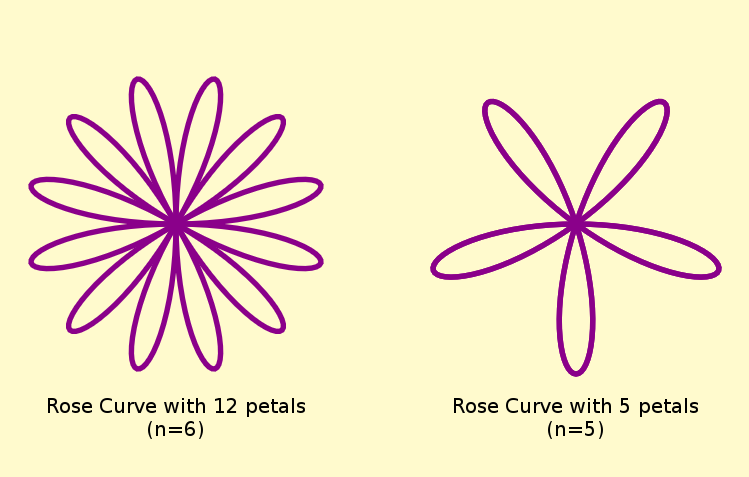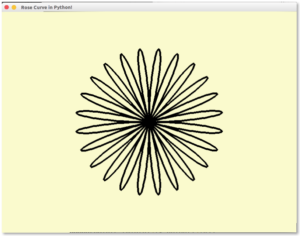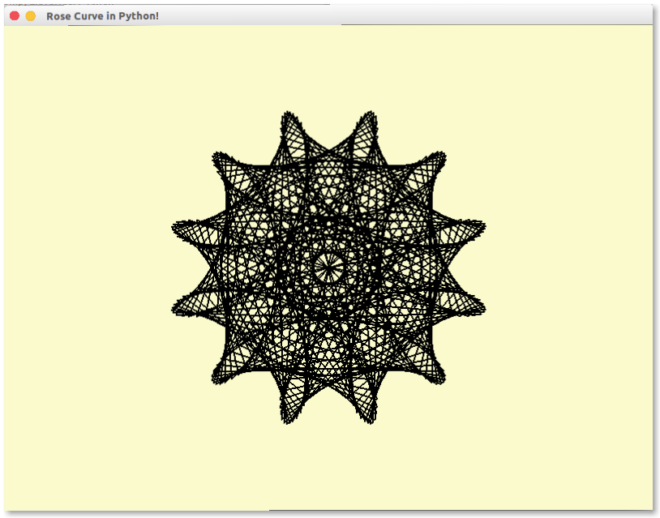# Rhodonea Curves and Maurer Rose in Python

In this article, we will create a Rhodonea Curve and Maurer Rose pattern in Python! Before we proceed to look at what exactly is a rhodonea curve or maurer rose we need to get the basic structure of our program ready!

## Basic Structure of the Program –

Before we move on to learn anything about Rhodonea curves or Maurer rose patterns, we first need to get the basic structure of our program ready. So that when we refactor our code we will only modify a function while the rest of the program will be the same. So here’s the basic structure of the program –

 from math import sin  from math import cos  from math import radians     import pygame     # The width and height of our program  (width, height) = (800, 600)     # Setup the window and init the screen surface  screen = pygame.display.set_mode((width, height))     pygame.display.set_caption('Rose Curve in Python !')     # The background color of the screen  screen.fill((250, 250, 205)) # lemonChiffon color     # Our function for drawing the rose pattern  drawPattern()     # Flip the drawn canvas with the newly created canvas (double-buffering)  # Basically we're refreshing the screen surface after drawing  pygame.display.flip()     # Our Main Loop  while True :                # Poll the events in the event queue      for event in pygame.event.get() :                # if the user closed the window           if event.type == pygame.QUIT :                  # deactivate pygame and quit the program              pygame.quit()                 quit()                # Draws the surface object to the screen.            pygame.display.update()

If you run the code, you will get an error that drawPattern is not defined. We’ll define it later. But for now just let it be and let’s move on to understand what are Rhodonea curves!

## What is a Rhodonea Curve?

Rhodonea Curve (also known as the rose in mathematics) is basically a curve which can be expressed in the formwhereis an integer defining the number of petals (ifis even andwhen odd)

Confused ? See this image comparing a rose with 12 petals versus a rose with 5 petals –Note that the coordinates in the equation above are in polar form. But since in computer graphics we express pixel locations in cartesian form rather than polar form, the curve has to re-described as a set of all pointseach in parametric equation form as shown below:## Getting our hands dirty with Rose Curves

The above was just the theory and in case you didn’t understand the concept completely you will after you see it in action. Just add the following in line 17 (right above where we invoked drawPattern):

 # Draws a rose with n petals and of radius size about size  def drawRhodoneaCurve(n, size):      points =[]      for i in range(0, 361):          # The equation of a rhodonea curve          r = size * sin(radians(n * i))             # Converting to cartesian co-ordinates          x = r * cos(radians(i))          y = r * sin(radians(i))             list.append(points, (width / 2 + x, height / 2 + y))         # Draws a set of line segments connected by set of vertices points      # Also don't close the path and draw it black and set the width to 5      pygame.draw.lines(screen, (0, 0, 0), False, points, 5)     def drawPattern():      # Try changing these values to what you want      drawRhodoneaCurve(12, 200)

This will produce the following result –Explanation

• We calculate the vertices (each significant point on the rose which are later connected by edges which are simply line-segments) using the formula we discussed in the above section
• We store a list called points which has all the vertices of the rose and later this list of vertices is feeded to pygame.draw.lines which draws a sequence of continuous straight lines and here we use it to draw polygonal chain (since a rose is just a polygonal chain)
• Note that we are using radians method here. This is because the angles are in degree format (we could have directly used radians but range expects only integers, also for maurer curves we’ll anyways have to use degrees so why not start from now) – hopefully we didn’t have to do any conversion ourselves as Python3 provides built-in functions degrees and radians.
• Also note that we are shifting the coordinates by (width/2, height/2). This is because by default PyGame has its coordinates in Top-Left corner. But we want it in the center so we shift it by half the screen width and height. We could have translated the whole coordinate system but it’s easier this way!

A note about making the program more interactive:

The program currently isn’t that interactive but you can make it interactive by perhaps increasing the number of petals by a fractal amount every frame and depending on how fast/slow you increment you can get pretty awesome results. Making the program interactive is out of scope (since that’d’ significantly increase the LOC count and perhaps also increase the complexity of the program and violate the basic structure that we created in the beginning) but I experimented a bit and here‘s the result of first creating a variable n and then incrementing it by 0.1 at every frame!

## Now What is a Maurer Rose?

Now that we know about Rhodonea Curves let’s proceed to Maurer Rose:-

A Maurer rose is a rhodonea curve of the formconsisting of 361 lines connected by 361 points – each of the formfor everyin the set, whereis an integer.

Varying values ofcan create varying types of roses even if they all have the same number of petals (meaning same value of)

Now the cartesian cordinates will be of the form:-## Getting our hands dirty with Maurer Roses

So to make something out of what we just learned, we over-write the previous drawPattern function with the new one:-

 # Draws a maurer rose with value n and d it's size about size  def drawMaurerRose(n, d, size):      points =[]      for i in range(0, 361):          # The equation of a maurer rose          k = i * d          r = size * sin(radians(n * k))             # Converting to cartesian co-ordinates          x = r * cos(radians(k))          y = r * sin(radians(k))             list.append(points, (width / 2 + x, height / 2 + y))         # Draws a set of line segments connected by set of vertices points      # Also don't close the path and draw it black and set the width to 5      pygame.draw.lines(screen, (0, 0, 0), False, points, 5)     def drawPattern():      # Try changing these values to what you want      drawMaurerRose(6, 79, 200)

And now when you run the program you should get the following result –Explanation:

Only the highlighted lines have been changed rest everything is same –

• The function name has been changed to drawMaurerRose from drawRhodoneaCurve and now expects an extra parameter d!
• We now introduce a new variable k which actually comes from the formula. It’s value at each iteration is equal to i*d, so for d=1 the function is exactly the same as drawRhodoneaCurve!!
• We now use k instead of i and it should be noted that the line width has been changed to 2 from 5 (otherwise some lines would appear as one because of their high thickness).
• And the invoke statement has ofcourse been changed as well, it now calls the newly created drawMaurerRose function with n=6 and d=71. The values were copied from WikiPedia since random value of d can sometimes produce weird looking roses!

References:

My Personal Notes arrow_drop_upCheck out this Author's contributed articles.

If you like GeeksforGeeks and would like to contribute, you can also write an article using contribute.geeksforgeeks.org or mail your article to contribute@geeksforgeeks.org. See your article appearing on the GeeksforGeeks main page and help other Geeks.

Please Improve this article if you find anything incorrect by clicking on the "Improve Article" button below.

Article Tags :

1

Please write to us at contribute@geeksforgeeks.org to report any issue with the above content.